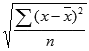The Excel STDEV.P function is a Statistical formula that calculates and returns the standard deviation for the entire population of data. The standard deviation is a measure of how much values are dispersed from the mean of a data set. The STDEV.P calculates the standard deviation using the "n" method. In this guide, we’re going to show you how to use the Excel STDEV.P function and also go over some tips and error handling methods.

## Supported versions

• Excel 2010 and later

## Excel STDEV.P Syntax

STDEV.P(number1,[number2],...)

## Arguments

 number1 The first number or reference in the population. You can also use an array instead of multiple arguments. [number2], … Other numbers of the population up to 254 arguments.

## Excel STDEV.P Use Cases

The standard deviation is a measure of how widely values are dispersed from the middle value (the mean). The Excel STDEV.P (STDEV P) function assumes that given arguments are the entire population. The function uses the following formula:where x is the average (mean) and n is the sample size.

All you need to do is to enter your dataset as the function arguments.

=STDEV.P(B5:B13)## Tips

• Use the Excel STDEV.P (STDEV P) to calculate the standard deviation for entire population.
• The standard deviation is calculated using the "n" method.
• Prefer using the STDEV.S to calculate the standard deviation for a sample of the population. The STDEV.S function uses the "n - 1" method.
• You can use the AVERAGE function to calculate the mean of a data set.
• The STDEVP is the older version of this function. We recommend refraining from using the STDEVP function.
• Arguments can be numbers or names, arrays, or references that contain numbers.
• Logical values, and text representations of numbers that you type directly into the list of arguments are counted.
• If an argument is an array or reference, only numbers in that array or reference are counted. Empty cells, logical values, text, or error values in the array or reference are ignored.
• Arguments that are error values or text that cannot be translated into numbers will give errors.
• If you want to include logical values and text representations of numbers in a reference as part of the calculation, use the STDEVPA function instead.